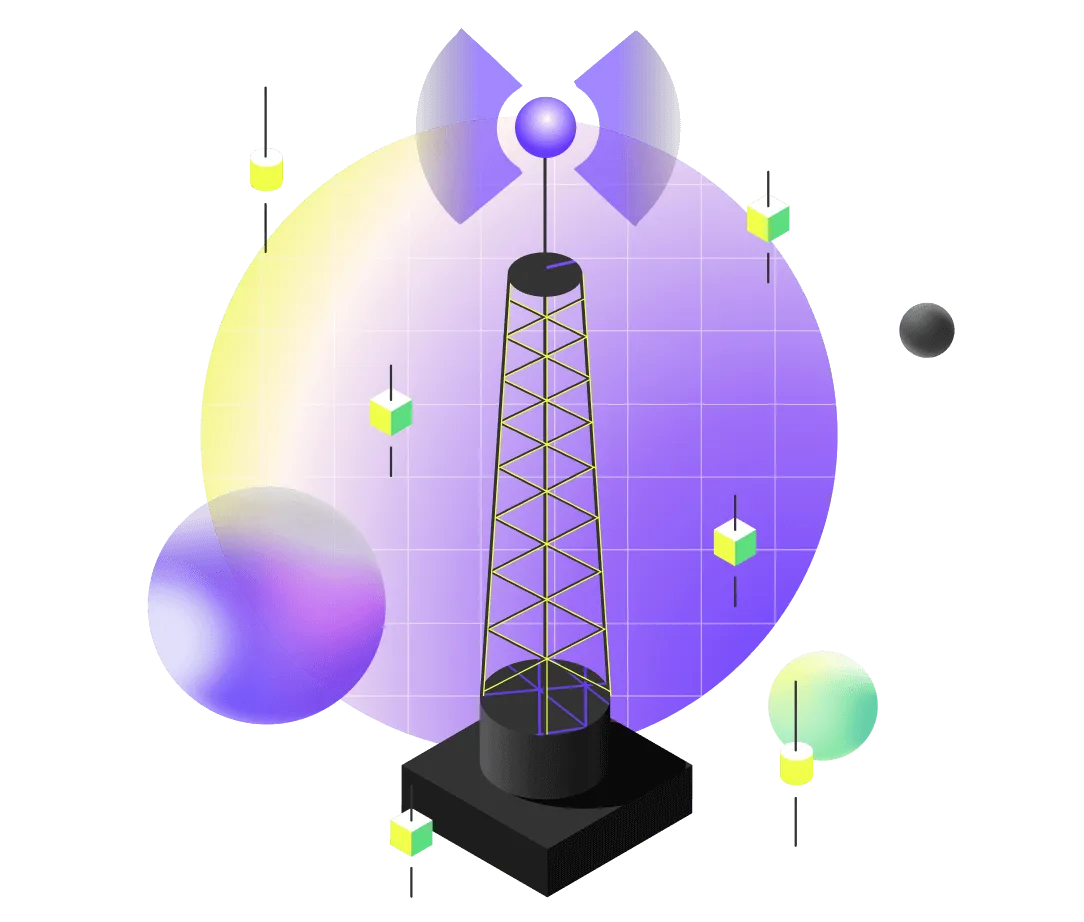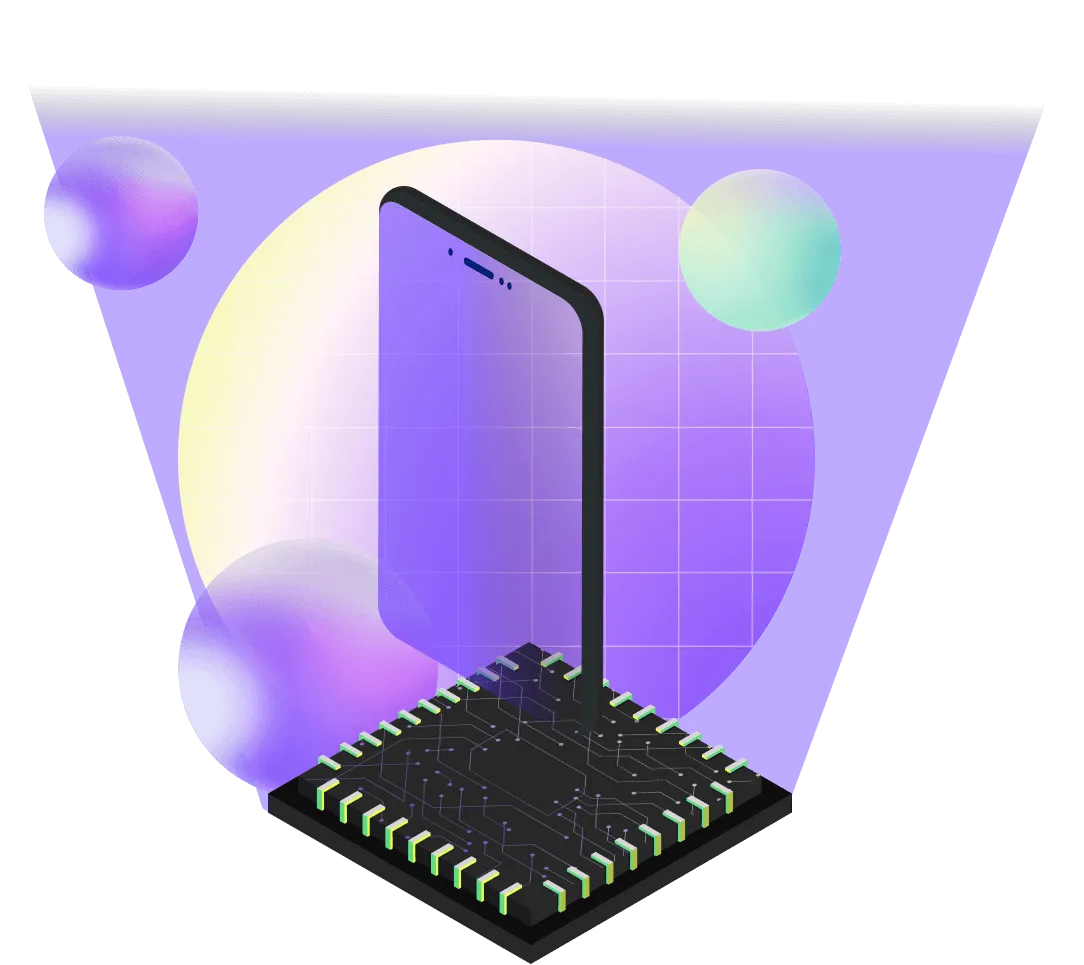# Quantum Optimization

QuEra's 256-qubit quantum computer can solve optimization problems relevant to a range of industries. One such optimization problem that maps naturally into our hardware is the Maximum Independent Set problem (MIS). The Maximum Independent Set of a graph is the largest possible subset of vertices such that none of the vertices are connected (e.g., share an edge). Finding such a set is an NP-hard problem. For general graphs, even approximate solutions may be beyond efficient analysis by classical algorithms. Many industrial problems can be formulated as an MIS problem such as footprint optimization for store locations (see this application note).

### Encoding a wide range of optimization problems

Solutions to MIS can give rise to solutions of other, related optimization problems, as described here. Using a range of algorithmic techniques, including quantum sampling, quantum memory encoding, quantum machine learning, variational optimization such as Quantum Adiabatic Algorithm (QAA) and Quantum Approximate Optimization Algorithm (QAOA), our machines can solve a range of other problems:Antenna placementMinimum vertex coverMinimum dominating setGraph coloring
The Weighted Maximum Independent Sets of a weighted graph can also be encoded on analog neutral atom quantum computers, by increasing the energy for certain atoms more than others. As described here, this gives rise to further optimization problemsInteger factorization# Building quantum computers from neutral-atom arrays### Finding the Maximum Independent Set

The Rydberg blockade effect is a quantum phenomenon that occurs when two closely situated neutral atoms are simultaneously excited to Rydberg states. This effect allows only one of the atoms to reach the excited state, and can be harnessed to find the Maximum Independent Set (MIS) of a graph. The process involves encoding the graph into an array of neutral atoms. The encoding is done in such a way that any two vertices of the graph that share an edge correspond to two atoms within the Rydberg blockade radius of each other. By raising the total energy in the system to a level high enough to excite the maximum number of atoms, the overall system state corresponds to a maximum independent set. The solution is then determined by measuring the state of the atoms - excited or not. The group of excited atoms represents the MIS.Image A: A maximum set of excited atoms, constrained by the Rydberg blockadeImage B: Highlighted in red: a maximum independent set of a graph (a maximum subset of vertices, so that none of the vertices share an edge).

#### An efficient solution

Solving the MIS problem on a neutral atom processor requires minimal overhead, as it takes advantage of the natural behavior of the atoms. If the graph of interest is a Unit Disk Graph (UDG), one can encode MIS problems with 256 variables on our 256 qubits. For certain graphs, analog neutral atom processors can find the MIS efficiently. A comparison with classical simulated annealing, a standard algorithm to solve optimization problems, shows that the neutral atom processor scales more favorable with the size of the problem for a precisely defined set of graphs. To learn more about the performance, read this publication of the result, or this blog post.### Industrial Applications of MIS

#### Antenna placement (Maximum Independent Set)

Maximizing the coverage of telecom services necessitates a high-density placement of antennas within a specific area. However, signal interference presents a challenge to this objective: coverage areas must not overlap, and minimum distance regulations between antennas are often imposed by governmental bodies. Among all potential location sites, the optimal antenna placements correspond to a maximum independent set, where no two coverage areas intersect. This problem can be expanded by maximizing 'weights' attached to each antenna, which could represent variables such as the total coverage area or the number of customers served. These principles can also be applied to related challenges, such as sensor placement for IoT applications or footprint optimization for the strategic location of stores or distribution centers.#### Portfolio optimization (Maximum clique)

In the financial industry, optimizing portfolios of assets, such as stocks or bonds, can enhance returns. Generally, Maximum Independent Sets (MISs) and their complement, maximum cliques, are valuable for deriving nontrivial insights into the correlation properties of large datasets. This information can guide strategies for hedging and diversification. One can construct a 'market graph,' where stocks correspond to vertices and vertices are connected when their correlations, θij, exceed a threshold, Θ. For instance, setting θij<Θ<0 and searching for graph cliques generates collections of mutually anti-correlated assets, maximizing returns while minimizing risk. Conversely, cliques obeying the limit θij>Θ>0 generate portfolios of positively-correlated assets whose prices move in tandem, as might occur for assets within the same market.#### 5G Networks (Minimum Dominating Set)

5G architectures are increasingly being utilized for device-to-device (D2D) communication networks, such as between cellphones or network-connected cars. This is particularly relevant in scenarios like a crowded concert or dense highway traffic. Nodes in these networks can communicate with each other using short-range protocols, like Bluetooth, as seen when adjacent autonomous cars exchange information. Alternatively, they can connect with the broader wireless network of cell towers, as when concert-goers upload pictures.
However, connecting every single node to the broader network of cell towers could potentially overload them. To mitigate this issue, a subset of nodes can be selected to serve as gateway nodes and only these are connected to the cell network. All other nodes in the area can then connect to the broader internet through a direct connection to an in-range gateway node, thereby reducing the cell tower bandwidth to a manageable level.### Get Started